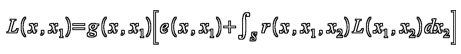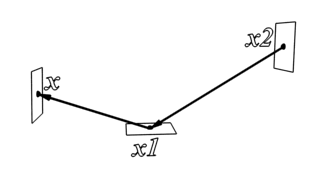The rendering equation

I. Exact vs approximate methods

II: Gathering vs shooting methods

III: Approximate methods: view-dependent vs view-independent solutions

GI methods supported by V-Ray

## The rendering equation

Virtually all modern GI renderers are based on the rendering equation introduced by James T. Kajiya in his 1986 paper "The Rendering Equation". This equation describes how light is propagated throughout a scene. In his paper, Kajiya also proposed a method for computing an image based on the rendering equation using a Monte Carlo method called path tracing.

It should be noted that the equation has been known long before that in engineering and has been used for computing radiative heat transfer in different environments. However, Kajiya was the first to apply this equation to computer graphics.

It should also be noted that the rendering equation is only "an approximation of Maxwell's equation for electromagnetics". It does not attempt to model all optical phenomena. It is only based on geometric optics and therefore cannot simulate things like diffraction, interference or polarization. However, it can be easily modified to account for wavelength-dependent effects like dispersion.

Another, more philosophical point to make, is that the rendering equation is derived from a mathematical model of how light behaves. While it is a very good model for the purposes of computer graphics, it does not describe exactly how light behaves in the real world. For example, the rendering equation assumes that light rays are infinitesimally thin and that the speed of light is infinite - neither of these assumptions is true in the real physical world.

Because the rendering equation is based on geometric optics, raytracing is a very convenient way to solve the rendering equation. Indeed, most renderers that solve the rendering equation are based on raytracing.

Different formulations of the rendering equation are possible, but the one proposed by Kajiya looks like this:where:

L(x, x1) is related to the light passing from point x1 to point x;

g(x, x1) is a geometry (or visibility term);

e(x, x1) is the intensity of emitted light from point x1 towards point x;

r(x, x1, x2) is related to the light scattered from point x2 to point x through point x1;

S is the union of all surfaces in the scene and x, x1 and x2 are points from S.

What the equation means: the light arriving at a given point x in the scene from another point x1 is the sum of the light emitted from all other points x2 towards x1 and reflected towards x:Except for very simple cases, the rendering equation cannot be solved exactly in a finite amount of time on a computer. However, we can get as close as we want to the real solution - given enough time. The search for global illumination algorithms has been a quest for finding solutions that are reasonably close, for a reasonable amount of time.

The rendering equation is only one. Different renderers only apply different methods for solving it. If any two renderers solve this equation accurately enough, then they should generate the same image for the same scene. This is very well in theory, but in practice renderers often truncate or alter parts of the rendering equation, which may lead to different results.

## I: Exact vs approximate methods

As noted above, we cannot solve the equation exactly - there is always some error, although it can be made very small. In some rendering methods, the desired error is specified in advance by the user and it determines the accuracy of the calculations (f.e. GI sample density, or GI rays, or number of photons etc.). A disadvantage of these methods is that the user must wait for the whole calculation process to complete before the result can be used. Another disadvantage is that it may take a lot of trials and errors to find settings that produce adequate quality in a given time frame. However, the big advantage of these methods is that they can be very efficient within the specified accuracy bounds, because the algorithm can concentrate on solving difficult parts of the rendering equation separately (e.g. splitting the image into independent regions, performing several calculation phases etc.), and then combining the result.

In other methods, the image is calculated progressively - in the beginning the error is large, but gets smaller as the algorithm performs additional calculations. At any one point of time, we have the partial result for the whole image. So, we can terminate the calculation and use the intermediate result.

### Exact (unbiased or brute-force) methods.

• Produce very accurate results.
• The only artifact these methods produce is noise.
• Renderers using exact methods typically have only few controls for specifying image quality.
• Typically require very little additional memory.

• Unbiased methods are not adaptive and so are extremely slow for a noiseless image.
• Some effects cannot be computed at all by an exact method (for example, caustics from a point light seen through a perfect mirror).
• It may be difficult to impose a quality requirement on these methods.
• Exact methods typically operate directly on the final image; the GI solution cannot be saved and re-used in any way.

Examples:

• Path tracing (brute-force GI in some rendereres).
• Bi-directional path tracing.
• Metropolis light transport.

### Approximate (biased) methods:

• Adaptive, so typically those are a lot faster than exact methods.
• Can compute some effects that are impossible for an exact method (e.g. caustics from a point light seen through a perfect mirror).
• Quality requirements may be set and the solution can be refined until those requirements are met.
• For some approximate methods, the GI solution can be saved and re-used.

• Results may not be entirely accurate (e.g. may be blurry) although typically the error can be made as small as necessary.
• Artifacts are possible (e.g. light leaks under thin walls etc).
• More settings for quality control.
• Some approximate methods may require (a lot of) additional memory.

Examples:

• Photon mapping.
• Light cache in V-Ray.

### Hybrid methods: exact methods used for some effects, approximate methods for others.

• Combine both speed and quality.

• May be more complicated to set up.

Examples:

• Final gathering with Min/Max radius 0/0 + photon mapping in mental ray.
• brute force GI + photon mapping or light cache in V-Ray.
• Light tracer with Min/Max rate 0/0 + radiosity in 3ds Max.
• Some methods can be asymptotically unbiased - that is, they start with some bias initially, but it is gradually decreased as the calculation progresses.

## II: Gathering vs shooting methods

### Shooting methods

These start from the lights and distribute light energy throughout the scene. Note that shooting methods can be either exact or approximate.

• Can easily simulate some specific light effects like caustics.

• They don't take into consideration the camera view; thus they might spend a lot of time for parts of the scene that are not visible or do not contribute to the image (e.g. caustics that are not visible - they must still be computed).
• Produce more precise solutions for portions of the scene that are close to lights; regions that are far from light sources may be computed with insufficient precision.
• Cannot simulate efficiently all kinds of light effects e.g. object lights and environment lights (skylight); non-physical light sources are difficult to simulate.

Examples:

• photon mapping (approximate).
• particle tracing (approximate).
• light tracing (exact).

### Gathering methods

These start from the camera and/or the scene geometry. Note that gathering methods can be either exact or approximate.

• They work based on which parts of the scene we are interested in; therefore, they can be more efficient than shooting methods.
• Can produce a very precise solution for all visible parts of the image.
• Can simulate various light effects (object and environment lights), non-physical lights.

• Some light effects (caustics from point lights or small area lights) are difficult or impossible to simulate.

Examples

• path tracing (exact)
• irradiance caching (final gathering in mental ray), (approximate).

### Hybrid methods

These combine shooting and gathering; again, hybrid methods can be either exact or approximate.

• Can simulate nearly all kinds of light effects

• May be difficult to implement and/or set up.

Examples:

• final gathering + photon mapping in mental ray (approximate).
• irradiance map/brute force GI + photon map in V-Ray (approximate).
• bi-directional path tracing and metropolis light transport (exact).

## III: Approximate methods: view-dependent vs view-independent solutions

Some approximate methods allow the caching of the GI solution. The cache can be either view-dependent or view-independent.

### Shooting methods

• Shooting methods typically produce a view-independent solution.

• The solution is typically of low quality (blurry and lacking details). Detailed solution requires a lot of time and/or memory.
• Adaptive solutions are difficult to produce.
• Regions that are far from light sources may be computed with insufficient accuracy.

Examples:

• photon mapping

### Gathering methods

Gathering methods and some hybrid methods allow for both view-dependent and view-independent solutions.

#### View-dependent solutions

• Only the relevant parts of the scene are taken into consideration (no time is wasted on regions that are not visible).
• Can work with any kind of geometry (i.e. no restriction on geometry type).
• Can produce very high-quality results (keeping all the fine details).
• In some methods, view-dependent portions of the solution can be cached as well (glossy reflections, refractions etc).
• Require less memory than a view-independent solution.

• Requires updating for different camera positions; still, in some implementations portions of the solution may be re-used.

Examples:

• Irradiance caching (in V-Ray, mental ray, finalRender, Brazil r/s, 3ds Max's light tracer).

#### View-independent solutions

• Solution needs to be computed only once.

• All of the scene geometry must be considered, even though some of it may never be visible.
• The type of geometry in the scene is usually restricted to trianglular or quadrangular meshes (no procedural or infinite geometry allowed).
• Detailed solutions require lots of memory.
• Only the diffuse portion of the solution can be cached; view-dependent portions (glossy reflections) must still be computed.

Examples:

### Hybrid methods

Different combinations of view-dependent and view-independent techniques can be combined.

Examples:

• photon mapping and irradiance caching in V-Ray.
• photon mapping and final gathering in mental ray.
• radiosity and light tracer in 3ds Max.

## GI methods supported by V-Ray

V-Ray supports a number of different methods for solving the GI equation - exact, approximate, shooting and gathering. Some methods are more suitable for some specific types of scenes.

### Exact methods

V-Ray supports two exact methods for calculating the rendering equation: brute force GI and progressive path tracing. The difference between the two is that brute force GI works with traditional image construction algorithms (bucket rendering) and is adaptive, whereas path tracing refines the whole image at once and does not perform any adaptation.

### Approximate methods

All other methods used V-Ray (irradiance map, light cache, photon map) are approximate methods.

### Shooting methods

The photon map is the only shooting method in V-Ray. Caustics can also be computed with photon mapping, in combination with a gathering method.

### Gathering methods

All other methods in V-Ray (brute force GI, irradiance map, light cache) are gathering methods.

### Hybrid methods

V-Ray can use different GI engines for primary and secondary bounces, which allows you to combine exact and approximate, shooting and gathering algorithms, depending on what is your goal. Some of the possible combinations are demonstrated on the GI examples page.

Хостинг от uCoz